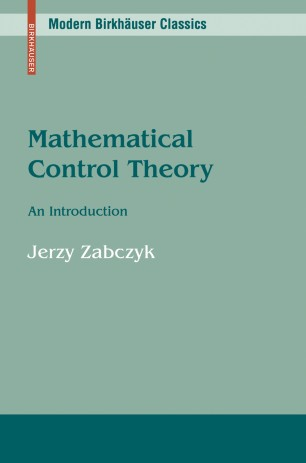# Mathematical Control Theory

## An Introduction

• Jerzy ZabczykTextbook

Part of the Modern Birkhäuser Classics book series (MBC)

1. Front Matter
Pages i-x
2. ### Introduction

1. Jerzy Zabczyk
Pages 1-9
3. ### Elements of classical control theory

1. Jerzy Zabczyk
Pages 10-27
2. Jerzy Zabczyk
Pages 28-49
3. Jerzy Zabczyk
Pages 50-61
4. Jerzy Zabczyk
Pages 62-72
4. ### Nonlinear control systems

1. Jerzy Zabczyk
Pages 73-91
2. Jerzy Zabczyk
Pages 92-120
3. Jerzy Zabczyk
Pages 121-126
5. ### Optimal control

1. Jerzy Zabczyk
Pages 127-141
2. Jerzy Zabczyk
Pages 142-151
3. Jerzy Zabczyk
Pages 152-169
4. Jerzy Zabczyk
Pages 170-175
6. ### Infinite dimensional linear systems

1. Jerzy Zabczyk
Pages 176-205
2. Jerzy Zabczyk
Pages 206-220
3. Jerzy Zabczyk
Pages 221-231
4. Jerzy Zabczyk
Pages 232-243
7. Back Matter
Pages 244-260

### Introduction

Mathematical Control Theory: An Introduction presents, in a mathematically precise manner, a unified introduction to deterministic control theory. With the exception of a few more advanced concepts required for the final part of the book, the presentation requires only a knowledge of basic facts from linear algebra, differential equations, and calculus.

In addition to classical concepts and ideas, the author covers the stabilization of nonlinear systems using topological methods, realization theory for nonlinear systems, impulsive control and positive systems, the control of rigid bodies, the stabilization of infinite dimensional systems, and the solution of minimum energy problems.

The book will be ideal for a beginning graduate course in mathematical control theory, or for self study by professionals needing a complete picture of the mathematical theory that underlies the applications of control theory.

"This book is designed as a graduate text on the mathematical theory of deterministic control. It covers a remarkable number of topics...The exposition is excellent, and the book is a joy to read. A novel one-semester course covering both linear and nonlinear systems could be given...The book is an excellent one for introducing a mathematician to control theory."   Bulletin of the AMS

"The book is very well written from a mathematical point of view of control theory. The author deserves much credit for bringing out such a book which is a useful and welcome addition to books on the mathematics of control theory."   — Control Theory and Advance Technology

"At last! We did need an introductory textbook on control which can be read, understood, and enjoyed by anyone."   — Gian-Carlo Rota, The Bulletin of Mathematics Books

### Keywords

control control system control theory dynamic programming infinite dimensional linear systems linear systems mathematical control theory nonlinear control nonlinear system observability optimal control programming stability stabilization sys

#### Authors and affiliations

• Jerzy Zabczyk
• 1
1. 1.Institute of MathematicsPolish Academy of SciencesWarsawPoland

Industry Sectors